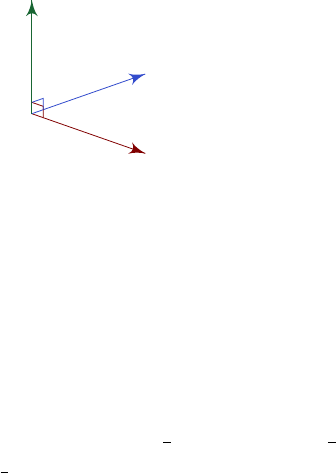2Vectors

IA Vectors and Matrices2.4 Vector product
Apart from the scalar product, we can also define the vector product. However,
this is defined only for R
3
space, but not spaces in general.
Definition
(Vector/cross product)
.
Consider
a, b R
3
. Define the vector
product
a × b = |a||b|sin θ
ˆ
n,
where
ˆn
is a unit vector perpendicular to both
a
and
b
. Since there are two
(opposite) unit vectors that are perpendicular to both of them, we pick
ˆn
to be
the one that is perpendicular to a, b in a right-handed sense.
a
b
a × b
The vector product satisfies the following properties:
(i) a × b = b × a.
(ii) a × a = 0.
(iii) a × b = 0 a = λb for some λ R (or b = 0).
(iv) a × (λb) = λ(a × b).
(v) a × (b + c) = a × b + a × c.
If we have a triangle
OAB
, its area is given by
1
2
|
OA||
OB|sin θ
=
1
2
|
OA×
OB|
.
We define the vector area as
1
2
OA ×
OB
, which is often a helpful notion when
we want to do calculus with surfaces.
There is a convenient way of calculating vector products:
Proposition.
a × b = (a
1
ˆ
i + a
2
ˆ
j + a
3
ˆ
k) × (b
1
ˆ
i + b
2
ˆ
j + b
3
ˆ
k)
= (a
2
b
3
a
3
b
2
)
ˆ
i + ···
=
ˆ
i
ˆ
j
ˆ
k
a
1
a
2
a
3
b
1
b
2
b
3